# Keras TensorFlow教程：如何从零开发一个复杂深度学习模型Keras 是提供一些高可用的 Python API ，能帮助你快速的构建和训练自己的深度学习模型，它的后端是 TensorFlow 或者 Theano 。本文假设你已经熟悉了 TensorFlow 和卷积神经网络，如果，你还没有熟悉，那么可以先看看这个10分钟入门 TensorFlow 教程和卷积神经网络教程，然后再回来阅读这个文章。

17为什么选择 Keras？为什么 Keras 被认为是深度学习的未来？

18在Ubuntu上面一步一步安装Keras。

19Keras TensorFlow教程：Keras基础知识。

20了解 Keras 序列模型

4.1 实际例子讲解线性回归问题

21使用 Keras 保存和回复预训练的模型

22Keras API

6.1 使用Keras API开发VGG卷积神经网络

6.2 使用Keras API构建并运行SqueezeNet卷积神经网络

1. 为什么选择Keras？

Keras 是 Google 的一位工程师François Chollet 开发的一个框架，可以帮助你在 Theano 上面进行快速原型开发。后来，这被扩展为 TensorFlow 也可以作为后端。并且最近，TensorFlow决定将其作为 contrib 文件中的一部分进行提供。

Keras 被认为是构建神经网络的未来，以下是一些它流行的原因：

23轻量级和快速开发：Keras 的目的是在消除样板代码。几行 Keras 代码就能比原生的 TensorFlow 代码实现更多的功能。你也可以很轻松的实现 CNN 和 RNN，并且让它们运行在 CPU 或者 GPU 上面。

24框架的“赢者”：Keras 是一个API，运行在别的深度学习框架上面。这个框架可以是 TensorFlow 或者 Theano。Microsoft 也计划让 CNTK 作为 Keras 的一个后端。目前，神经网络框架世界是非常分散的，并且发展非常快。具体，你可以看看 Karpathy 的这个推文：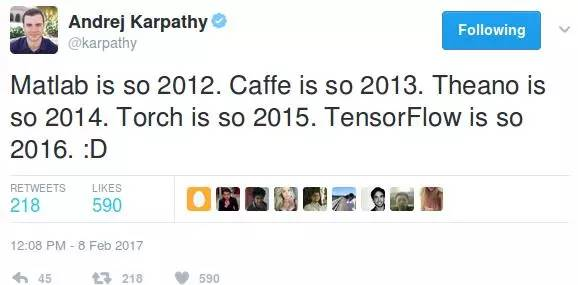2. 怎么安装Keras，并且把TensorFlow作为后端

a) 依赖安装

pip install h5py

pip install numpy scipy

pip install pillow

sudo pip install keras

>>> import keras

Using TensorFlow backend.

>>> keras.__version__

'2.0.4'

{

"floatx": "float32",

"epsilon": 1e-07,

"backend": "tensorflow",

"image_data_format": "channels_last"

}

3. Keras基础知识

import keras

Keras 有两种不同的建模方式：

Sequential models：这种方法用于实现一些简单的模型。你只需要向一些存在的模型中添加层就行了。

Functional API：Keras的API是非常强大的，你可以利用这些API来构造更加复杂的模型，比如多输出模型，有向无环图等等。

4. Keras Sequential models

from keras.models import Sequential

models = Sequential()

from keras.layers import Dense, Activation, Conv2D, MaxPooling2D, Flatten, Dropout

model.add(Conv2D(64, (3,3), activation='relu', input_shape = (100,100,32)))

# This ads a Convolutional layer with 64 filters of size 3 * 3 to the graph

model.add(Conv2D(64, (3,3), activation='relu', input_shape = (100,100,32)))

MaxPooling 层

model.add(MaxPooling2D(pool_size=(2,2)))

model.add(Dense(256, activation='relu'))

Dropout

model.add(Dropout(0.5))

model.add(Flatten())

model.add(Conv2D(32, (3,3), activation='relu', input_shape=(224, 224, 3)))

model.compile(loss='binary_crossentropy', optimizer='rmsprop')

from keras.optimizers import SGD

sgd = SGD(lr=0.01, decay=1e-6, momentum=0.9, nesterov=True)

model.compile(loss='categorical_crossentropy', optimizer=sgd)

model.fit(x_train, y_train, batch_size = 32, epochs = 10, validation_data(x_val, y_val))

score = model.evaluate(x_test, y_test, batch_size = 32)

4.1 实际例子讲解线性回归问题

a) 创建训练数据

TrainX 的数据范围是 -1 到 1，TrainY 与 TrainX 的关系是3倍，并且我们加入了一些噪声点。

import keras

from keras.models import Sequential

from keras.layers import Dense

import numpy as np

trX = np.linspace(-1, 1, 101)

trY = 3 * trX + np.random.randn(*trX.shape) * 0.33

b) 构建模型

model = Sequential()

model.add(Dense(input_dim=1, output_dim=1, init='uniform', activation='linear'))

weights = model.layers.get_weights()

w_init = weights

b_init = weights

print('Linear regression model is initialized with weights w: %.2f, b: %.2f' % (w_init, b_init))

## Linear regression model is initialized with weight w: -0.03, b: 0.00

model.compile(optimizer='sgd', loss='mse')

model.fit(trX, trY, nb_epoch=200, verbose=1)

weights = model.layers.get_weights()

w_final = weights

b_final = weights

print('Linear regression model is trained to have weight w: %.2f, b: %.2f' % (w_final, b_final))

##Linear regression model is trained to have weight w: 2.94, b: 0.08

5. 使用 Keras 保存和回复预训练的模型

HDF5 二进制格式

model.save_weights("my_model.h5")

model.load_weights('my_model_weights.h5')

6. Keras API

from keras.models import Model

from keras.layers import Input## First, define the vision modulesdigit_input = Input(shape=(1, 28, 28))

from keras.layers import Input

## First, define the vision modules

digit_input = Input(shape=(1, 28, 28))

vision_model = Model(digit_input, out)

6.1 使用Keras API开发VGG卷积神经网络

VGG：VGG卷积神经网络是牛津大学在2014年提出来的模型。当这个模型被提出时，由于它的简洁性和实用性，马上成为了当时最流行的卷积神经网络模型。它在图像分类和目标检测任务中都表现出非常好的结果。在2014年的ILSVRC比赛中，VGG 在Top-5中取得了92.3%的正确率。 该模型有一些变种，其中最受欢迎的当然是 vgg-16，这是一个拥有16层的模型。你可以看到它需要维度是 224*224*3 的输入数据。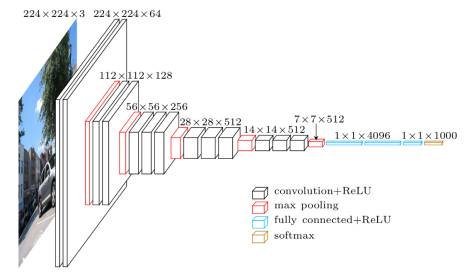Vgg 16 architecture

img_input = Input(shape=input_shape)

# Block 1

x = Conv2D(64, (3, 3), activation='relu', padding='same', name='block1_conv1')(img_input)

x = Conv2D(64, (3, 3), activation='relu', padding='same', name='block1_conv2')(x)

x = MaxPooling2D((2, 2), strides=(2, 2), name='block1_pool')(x)

# Block 2

x = Conv2D(128, (3, 3), activation='relu', padding='same', name='block2_conv1')(x)

x = Conv2D(128, (3, 3), activation='relu', padding='same', name='block2_conv2')(x)

x = MaxPooling2D((2, 2), strides=(2, 2), name='block2_pool')(x)

# Block 3

x = Conv2D(256, (3, 3), activation='relu', padding='same', name='block3_conv1')(x)

x = Conv2D(256, (3, 3), activation='relu', padding='same', name='block3_conv2')(x)

x = Conv2D(256, (3, 3), activation='relu', padding='same', name='block3_conv3')(x)

x = MaxPooling2D((2, 2), strides=(2, 2), name='block3_pool')(x)

# Block 4

x = Conv2D(512, (3, 3), activation='relu', padding='same', name='block4_conv1')(x)

x = Conv2D(512, (3, 3), activation='relu', padding='same', name='block4_conv2')(x)

x = Conv2D(512, (3, 3), activation='relu', padding='same', name='block4_conv3')(x)

x = MaxPooling2D((2, 2), strides=(2, 2), name='block4_pool')(x)

# Block 5

x = Conv2D(512, (3, 3), activation='relu', padding='same', name='block5_conv1')(x)

x = Conv2D(512, (3, 3), activation='relu', padding='same', name='block5_conv2')(x)

x = Conv2D(512, (3, 3), activation='relu', padding='same', name='block5_conv3')(x)

x = MaxPooling2D((2, 2), strides=(2, 2), name='block5_pool')(x)

x = Flatten(name='flatten')(x)

x = Dense(4096, activation='relu', name='fc1')(x)

x = Dense(4096, activation='relu', name='fc2')(x)

x = Dense(classes, activation='softmax', name='predictions')(x)

model = applications.VGG16(weights='imagenet')

img = image.load_img('cat.jpeg', target_size=(224, 224))

x = image.img_to_array(img)

x = np.expand_dims(x, axis=0)

x = preprocess_input(x)

preds = model.predict(x)

for results in decode_predictions(preds):

for result in results:

print('Probability %0.2f%% => [%s]' % (100*result, result))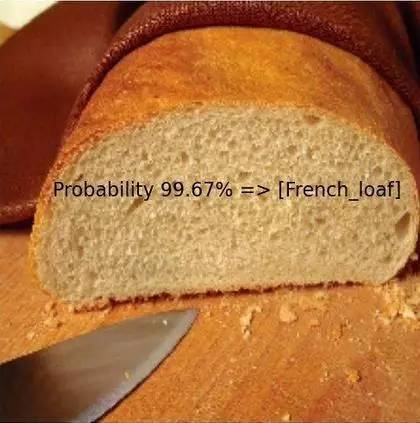6.2 使用Keras API构建并运行SqueezeNet卷积神经网络

SequeezeNet 是一个非常了不起的网络架构，它的显著点不在于对正确性有多少的提高，而是减少了计算量。当SequeezeNet的正确性和AlexNet接近时，但是ImageNet上面的预训练模型的存储量小于5 MB，这对于在现实世界中使用CNN是非常有利的。SqueezeNet模型引入了一个 Fire模型，它由交替的 Squeeze 和 Expand 模块组成。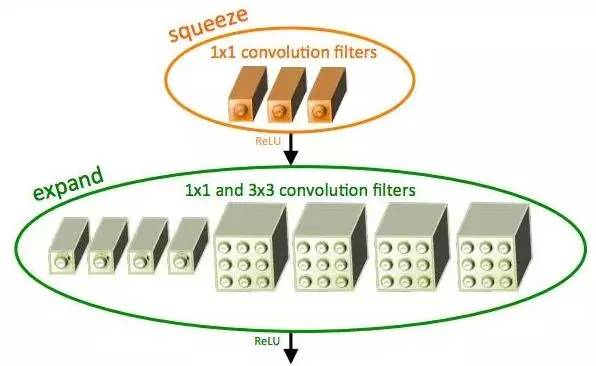SqueezeNet fire module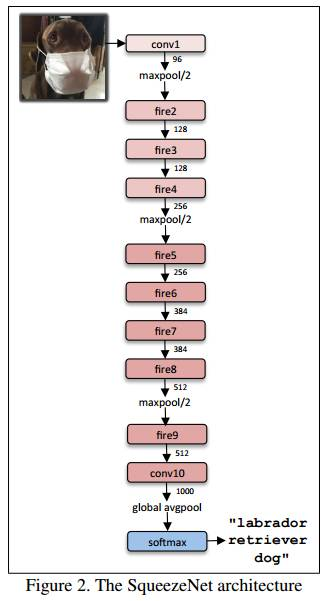# Squeeze part of fire module with 1 * 1 convolutions, followed by Relu

x = Convolution2D(squeeze, (1, 1), padding='valid', name='fire2/squeeze1x1')(x)

x = Activation('relu', name='fire2/relu_squeeze1x1')(x)

#Expand part has two portions, left uses 1 * 1 convolutions and is called expand1x1

left = Convolution2D(expand, (1, 1), padding='valid', name='fire2/expand1x1')(x)

left = Activation('relu', name='fire2/relu_expand1x1')(left)

#Right part uses 3 * 3 convolutions and is called expand3x3, both of these are follow#ed by Relu layer, Note that both receive x as input as designed.

right = Convolution2D(expand, (3, 3), padding='same', name='fire2/expand3x3')(x)

right = Activation('relu', name='fire2/relu_expand3x3')(right)

# Final output of Fire Module is concatenation of left and right.

x = concatenate([left, right], axis=3, name='fire2/concat')

sq1x1 = "squeeze1x1"

exp1x1 = "expand1x1"

exp3x3 = "expand3x3"

relu = "relu_"

WEIGHTS_PATH = "https://github.com/rcmalli/keras-squeezenet/releases/download/v1.0/squeezenet_weights_tf_dim_ordering_tf_kernels.h5"

sq1x1 = "squeeze1x1"

exp1x1 = "expand1x1"

exp3x3 = "expand3x3"

relu = "relu_"

def fire_module(x, fire_id, squeeze=16, expand=64):

s_id = 'fire' + str(fire_id) + '/'

x = Convolution2D(squeeze, (1, 1), padding='valid', name=s_id + sq1x1)(x)

x = Activation('relu', name=s_id + relu + sq1x1)(x)

left = Convolution2D(expand, (1, 1), padding='valid', name=s_id + exp1x1)(x)

left = Activation('relu', name=s_id + relu + exp1x1)(left)

right = Convolution2D(expand, (3, 3), padding='same', name=s_id + exp3x3)(x)

right = Activation('relu', name=s_id + relu + exp3x3)(right)

x = concatenate([left, right], axis=3, name=s_id + 'concat')

return x

x = Convolution2D(64, (3, 3), strides=(2, 2), padding='valid', name='conv1')(img_input)

x = Activation('relu', name='relu_conv1')(x)

x = MaxPooling2D(pool_size=(3, 3), strides=(2, 2), name='pool1')(x)

x = fire_module(x, fire_id=2, squeeze=16, expand=64)

x = fire_module(x, fire_id=3, squeeze=16, expand=64)

x = MaxPooling2D(pool_size=(3, 3), strides=(2, 2), name='pool3')(x)

x = fire_module(x, fire_id=4, squeeze=32, expand=128)

x = fire_module(x, fire_id=5, squeeze=32, expand=128)

x = MaxPooling2D(pool_size=(3, 3), strides=(2, 2), name='pool5')(x)

x = fire_module(x, fire_id=6, squeeze=48, expand=192)

x = fire_module(x, fire_id=7, squeeze=48, expand=192)

x = fire_module(x, fire_id=8, squeeze=64, expand=256)

x = fire_module(x, fire_id=9, squeeze=64, expand=256)

x = Dropout(0.5, name='drop9')(x)

x = Convolution2D(classes, (1, 1), padding='valid', name='conv10')(x)

x = Activation('relu', name='relu_conv10')(x)

x = GlobalAveragePooling2D()(x)

out = Activation('softmax', name='loss')(x)

model = Model(inputs, out, name='squeezenet')

import numpy as np

from keras_squeezenet import SqueezeNet

from keras.applications.imagenet_utils import preprocess_input, decode_predictions

from keras.preprocessing import image

model = SqueezeNet()

img = image.load_img('pexels-photo-280207.jpeg', target_size=(227, 227))

x = image.img_to_array(img)

x = np.expand_dims(x, axis=0)

x = preprocess_input(x)

preds = model.predict(x)

all_results = decode_predictions(preds)

for results in all_results:

for result in results:

print('Probability %0.2f%% => [%s]' % (100*result, result))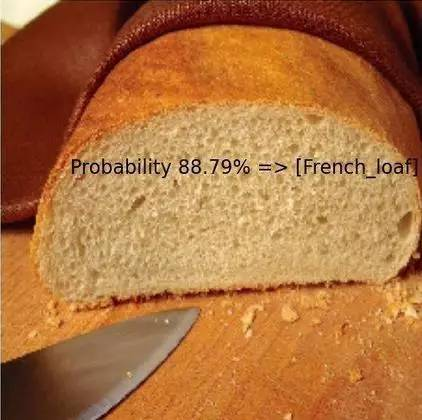12-271万+07-313214
01-09144
10-124万+
10-299562
08-23239
08-25318
10-31908
01-03865
11-1335
04-30140
08-19599
03-105万+
10-19
07-074513
09-2444
06-042559
02-195194
©️2020 CSDN 皮肤主题: 大白 设计师:CSDN官方博客